# O Level Exam

#### Continue Statement

Continue statement unlike the break statement is used to skip the remaining part of a loop and start with next iteration.

Syntax:

`continue`The working of continue statement in for and while loop is shown below.Example : Program to illustrate the use of continue statement inside for loop

`for word in “Jump Statement”:	if word = = “e”:		continue		print (word, end=”)print (“\n End of the program”)`

Output:

Jump Statement

End of the program

The above program is same as the program we had written for ‘break’ statement except that we have replaced it with ‘continue’. As you can see in the output except the letter ‘e’ all the other letters get printed.

Qus. What are operators? What is their function? Give examples of some unary and binary operators.

Ans: “Operators are those symbols used with operands, which tells compiler which operation is to be done on operands.‖ in other words – ―operators are tokens that trigger some computation/action when applied to variables and other objects in an expression.‖

Operators are of following types:

•  Unary operators like (+) Unary Plus, (-) Unary Minus, not etc.
•  Binary Operators like (+) addition, (*) multiplication, and etc.

Qus. What is block/code block/suit in Python?

Ans: Sometimes a group of statements is part of another statement of function. Such a group of one or more statements is called block or code-block or suit in python. e.g.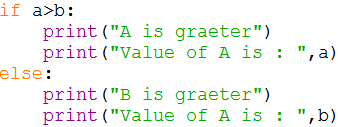Qus. What is the role of indentation in Python?

Ans: Indentation plays a very important role in Python. Python uses indentation to create blocks of code. Statements at same indentation level are part of same block/suit. You cannot unnecessarily indent a statement; python will raise an error for that.

Qus. How many types of strings are supported by Python?

Ans: Python supports two types of strings:

(i) Single-line string That terminates in single line.

(ii) Multi-line String That stores multiple lines of text.

Qus. What will be the sizes of following constants?

(a) "\a‟ (b) “\a” (c) “Kumar\‟s” (d) "\‟ (e) “it‟s”

Ans: (a) 50 (b) 50 (c) 56 (d) 50 (e) 53

This screenshot is the output of the above question.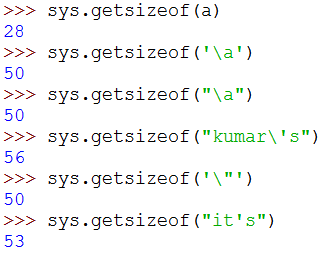Qus. How can you create multi-line strings in Python?

Ans: We can create multi-line string by putting a backslash (\) at the end of line which allows you to continue typing in next line in same string.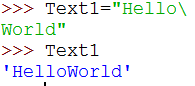Qus. Which of the following are syntactically correct strings? State reason.

(a) ”Python is nice Language”

(b) „He called me “Friend!” when he came‟

(c) “Very Good‟

(d) „This is a good book‟

(e) “Namaste

(f) “I liked „Harry Potter‟ very much”

Ans: (a) Correct (b) Correct (c) Incorrect (d) Correct (e) Incorrect (f) Correct

Qus. What is the error in following Python program with one statement?

print(“My name is : “, name)

suggest a solution

Ans: Error is : ―name 'name' is not defined‖. And the solution is to declare the variable-name before this statement.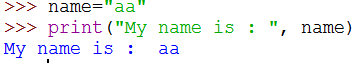Qus. Predict the output of the following: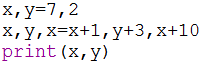Ans: Output: 17 5

Qus. What are augmented assignment operators? How are they useful?

Ans: An augmented assignment is generally used to replace a statement where an operator takes a variable as one of its arguments and then assigns the result back to the same variable. A simple example is x += 1 which is expanded to x = x + (1). Similar constructions are often available for various binary operators. They are helpful in making the source code small.

Qus. What are loops in Python? How many types of loop are there in Python?

Ans: Loops are iteration constructs in Python. Iteration means repetition of a set of statements depending upon a condition test. Loops has three basic elements within it to repeat the statements –

• Initialization (Start)
• Check Condition (Stop)
• Updation (Step)

Python provide two types of loop

(i) Conditional Loop while( (Condition based loop)

(ii) Counting loop for (loop for a given number of times).

Qus. What is the syntax of if-elif statement in Python?

Ans: The syntax of if-elif statement in python is as follows:

If condition1:

#code-block of statements when condition1 is true

elif condion2:

#code-block of statements when condition2 is true

elif condition3:

#code-block of statements when condition3 is true
.

.

.

else:

#code-block of statements when all above conditions are false.

Qus What are jump statements in Python? Name jump statements with example.

Ans: Python offers two jump statements to be used with in loops to jump out of loop-iterations.

These are break and continue statements.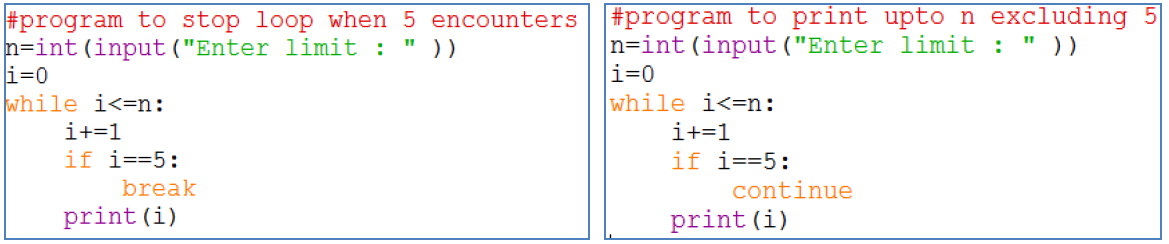#### Programs

Write a Program to obtain temperature in Celsius and convert it into Fahrenheit using formula –

C X 9/5 + 32 = F

WAP to read todays date (only date Part) from user. Then display how many days are left in the current month.

WAP to print the area of circle when radius of the circle is given by user.

WAP to print the volume of a cylinder when radius and height of the cylinder is given by user.

WAP that asks your height in centimeters and converts it into foot and inches.

WAP to accept 3 side of triangle and find area of a triangle.

WAP to input principle amount,rate time and calculate simple interest.

WAP to read a number in n and prints n^2, n^3, n^4

WAP to calculate compound simple interest after taking the principle, rate and time.

WAP to take two numbers and check that the first number is fully divisible by second number or not.

WAP to take value of x,y,z from the user and calculate the equation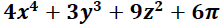WAP to take the temperatures of all 7 days of the week and displays the average temperature of that week.

WAP to check the given year is leap year or not.

WAP that searches for prime numbers from 15 through 25.

WAP to test if given number is prime or not.

WAP to compute the result when two numbers and one operator is given by user.

WAP to calculate the roots of a given quadratic equation.

WAP to input a digit and print it in words.

WAP to check whether square root of a given number is prime or not.

WAP to print first n odd numbers in descending order.

WAP to find the average of the list of the numbers entered through keyboard.

WAP to find the largest number from the list of the numbers entered through keyboard.

WAP to find the sum of n natural numbers.

WAP to find the sum of first n even numbers.

WAP to find the sum of first n odd numbers.

WAP a program to generate a list of elements of Fibonacci Series.

Program to find the simple interest based upon number of years. If number of years is more than 12 rate of interest is 10 otherwise 15.

Write a program to input any choice and to implement the following.

Choice Find

1. Area of square

2. Area of rectangle

3. Area of triangle

Write a program to input any number and to print all natural numbers up to given number.

Write a program to input any number and to find sum of all natural numbers up to given number.

Write a program to input any number and to find reverse of that number.

Write a program to input any string and count number of uppercase and lowercase letters.

Python program to obtain three numbers and print their sum.

Python program to obtain length and breath of a rectangle and calculate its area

Python program to calculate (BMI) body mass index of a person

Body Mass Index is a simple calculation using a person's height and weight. The formula is BMI=kg/m^2 where kg is a person's weight in kilogram and m^2 is the height in meter squared.

Write a program to input a number and print its cube.

Write a program to input two number and swap them.

Write a program to input three numbers and swap them as this : 1st number becomes the 2nd number, 2nd number becomes the 3rd number and 3rd number becomes the first number.

Write a program to read three numbers in three variables and swap first two variables with the sums of first and second ,second and third number respectively.

Write a program to input a single digit(n) and print a 3 digit number created as <n(n+1)(n+2)> e.g., if you input 7, then it should print 789. Assume that the input digit is in range 1-7.